# Linear Momentum, Angular Momentum and Kinetic Energy of Rigid Bodies (Part - 4) Civil Engineering (CE) Notes | EduRev

## Civil Engineering (CE) : Linear Momentum, Angular Momentum and Kinetic Energy of Rigid Bodies (Part - 4) Civil Engineering (CE) Notes | EduRev

The document Linear Momentum, Angular Momentum and Kinetic Energy of Rigid Bodies (Part - 4) Civil Engineering (CE) Notes | EduRev is a part of the Civil Engineering (CE) Course Introduction to Dynamics and Vibrations- Notes, Videos, MCQs.
All you need of Civil Engineering (CE) at this link: Civil Engineering (CE)

6.4.9 Time derivative of the inertia tensor

When we analyze motion of a rigid body, we will need to calculate the time derivatives of the linear and angular momentum.   Linear momentum is no problem, but for angular momentum, we will need to know how to differentiate IG with respect to time. There is a formula for this: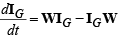where W = dR/dt RT is the spin tensor (see sect 6.2.2)

Proof:

• Start with IG = RIG0 RT and take the time derivative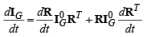• Recall that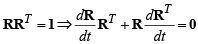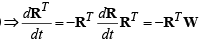• Finally note that dR / dt = WR and therefore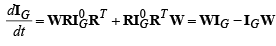6.4.9 Time derivative of angular momentum

To use the angular momentum conservation equation, we will need to know how to calculate the time derivative of the angular momentum.   When we do this for a 3D problem, we need to take into account that the mass moment of inertia changes as the body rotates.   We will prove the following formula:

dh/dt = rG × MaG +IGα + ω× IGω )

For 2D planar problems this can be simplified to:

dh/dt = rG × MaG + IGzzαz k

Proof: We start by taking the time derivative of the general definition of h

dh/dt = d/dt (rG x MvG +IGω )

We can go ahead and do the derivative with the product rule: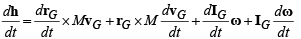We can simplify this by noting that drG / dt =vG and of course the cross product of vG with itself is zero. We can also use the definition of angular acceleration: dω / dt = α .   This gives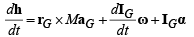Finally we can use the formula for dIG / dt from 6.4.8 to see that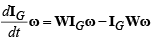and recalling the spin tensor-angular velocity formula Wu = ω ×u for all vectors u.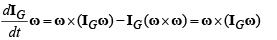where we recall that the cross product of a vector with itself is always zero.  This gives the answer stated.

Offer running on EduRev: Apply code STAYHOME200 to get INR 200 off on our premium plan EduRev Infinity!

## Introduction to Dynamics and Vibrations- Notes, Videos, MCQs

20 videos|53 docs

,

,

,

,

,

,

,

,

,

,

,

,

,

,

,

,

,

,

,

,

,

,

,

,

;